# Smooth Maximum¶

ReLU(x) = MAX(0, x)

S_\beta(x_1, ..., x_n) = \frac{\sum^n_{i=1}{x_i*e^{\beta*x_i}}}{\sum^n_{i=1}{e^{\beta*x_i}}}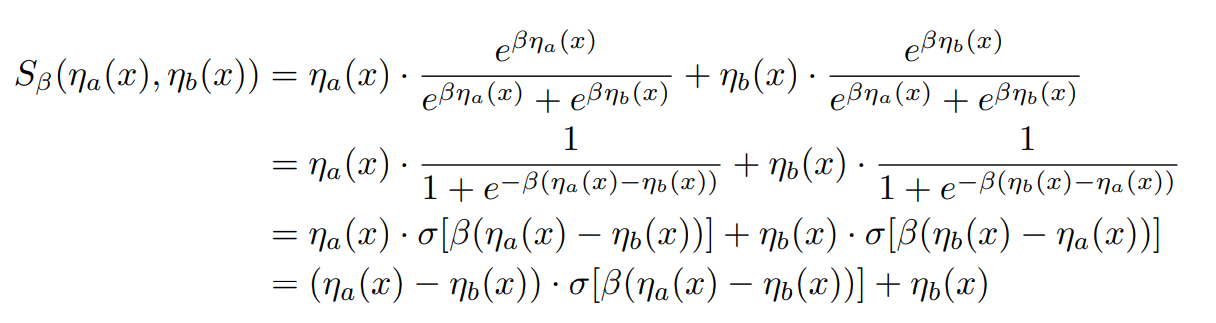import numpy as np
import matplotlib.pyplot as plt

def smooth_maximum(x, x1_func, x2_func, beta=0.0):
"""
Smooth Maximum
:param x: The input variable
:param x1_func: The functor of n1
:param x2_func: The functor of n2
:param beta: The beta value
:return:
"""
a_x = x1_func(x)
b_x = x2_func(x)

e_beta_a_x = np.exp(beta * a_x)
e_beta_b_x = np.exp(beta * b_x)

return ((a_x * e_beta_a_x) + (b_x * e_beta_b_x)) / (e_beta_a_x + e_beta_b_x)


# 从ReLU推广到Swish¶

S_\beta(0, x)

S_\beta(0, x) = x * \sigma(\beta*x)

def swish(x, beta=1.0):
return beta * x / (1 + np.exp(-x))

Acon_a = lambda x: smooth_maximum(x, x1_func=lambda x: x, x2_func=lambda x: 0, beta=1.0)

x = np.arange(-5, 5, 0.01).astype(np.float32)

acon_a_out = Acon_a(x)
swish_out = swish(x)

plt.plot(x, acon_a_out, ls='--')
plt.plot(x, swish_out, ls='-.')
plt.grid()
plt.show()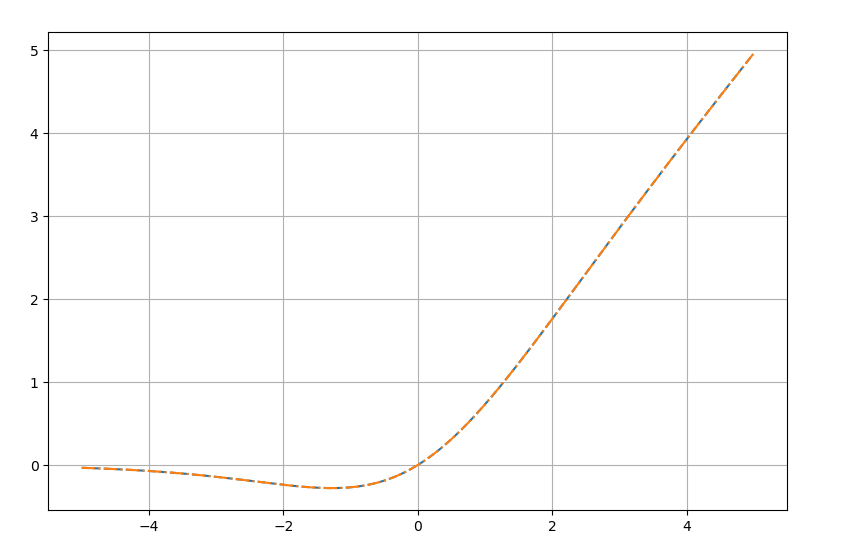# ACON-B¶

ACON-B(x)=S_{\beta}(x, p*x)=(1-p)*x *\sigma(\beta*(1-p)*x)+p*x

def leaky_relu(x, beta):
return np.maximum(x, beta * x)

Acon_b = lambda x: smooth_maximum(x, x1_func=lambda x: x, x2_func=lambda x: 0.2 * x, beta=1.0)

x = np.arange(-5, 5, 0.01).astype(np.float32)
acon_b_out = Acon_b(x)
leaky_relu_out = leaky_relu(x, 0.2)

plt.plot(x, acon_b_out)
plt.plot(x, leaky_relu_out)

plt.grid()
plt.show()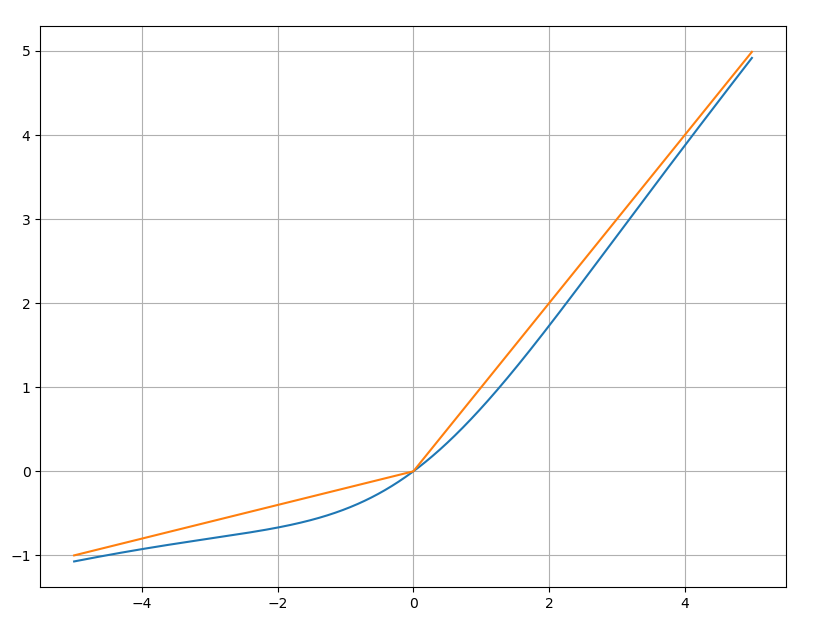# ACON-C¶

ACON-C(x)=S_{\beta}(p_1*x, p_2*x)=(p_1-p_2)*x*\sigma[\beta*(p_1-p_2)*x] + p_2*x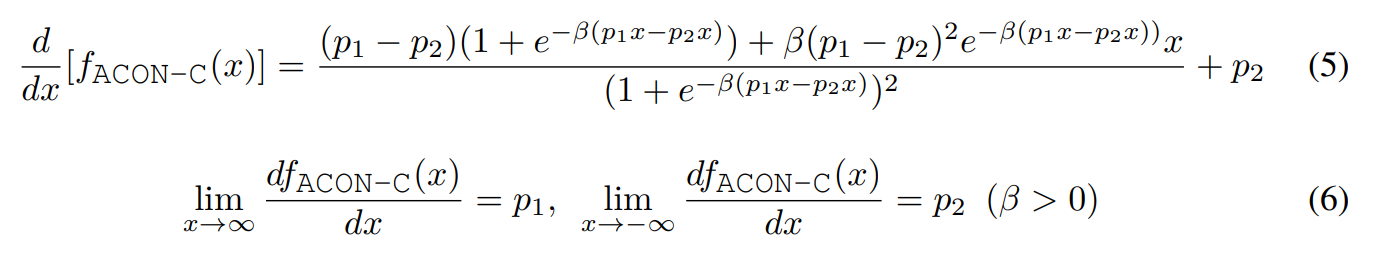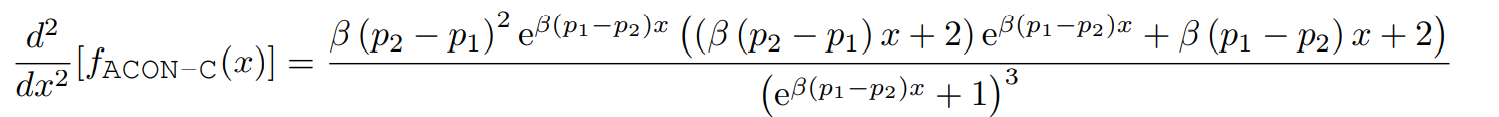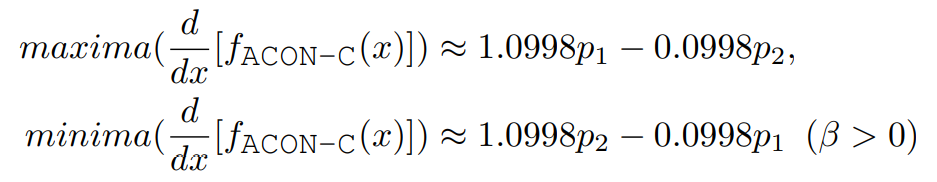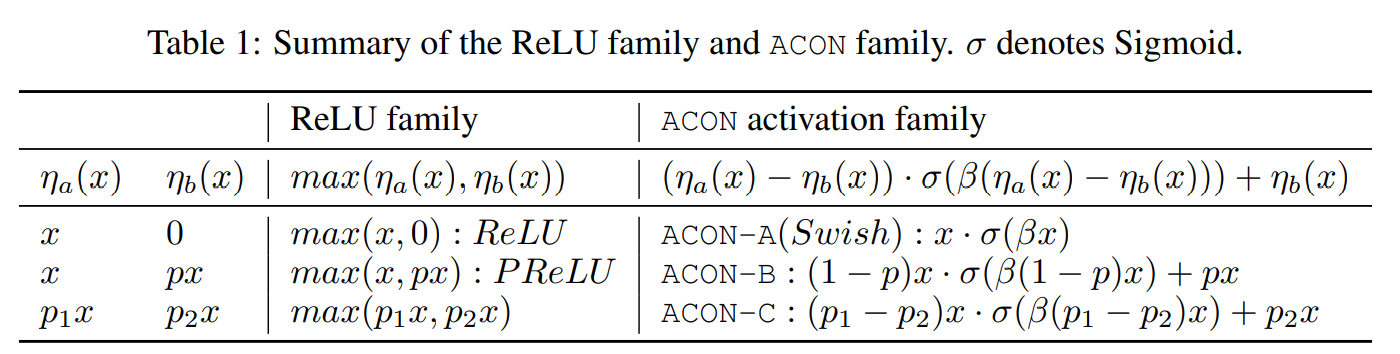# Meta-ACON¶

\beta_c=\sigma W_1W_2\sum^H_{h=1}\sum^W_{w=1}x_{c, h, w}

# 代码解读¶

import torch
from torch import nn

class MetaAconC(nn.Module):
r""" ACON activation (activate or not).
MetaAconC: (p1*x-p2*x) * sigmoid(beta*(p1*x-p2*x)) + p2*x
"""
def __init__(self, width):
super().__init__()
self.fc1 = nn.Conv2d(width, width//16, kernel_size=1, stride=1, bias=False)
self.fc2 = nn.Conv2d(width//16, width, kernel_size=1, stride=1, bias=False)

self.p1 = nn.Parameter(torch.randn(1, width, 1, 1))
self.p2 = nn.Parameter(torch.randn(1, width, 1, 1))

self.sigmoid = nn.Sigmoid()

def forward(self, x, **kwargs):
beta = self.sigmoid(self.fc2(self.fc1(x.mean(dim=2, keepdims=True).mean(dim=3, keepdims=True))))
return (self.p1 * x - self.p2 * x) * self.sigmoid( beta * (self.p1 * x - self.p2 * x)) + self.p2 * x


# 实验¶Meta-ACON虽然带来了一定的参数量，但是对大网络和小网络上都是有一定的提升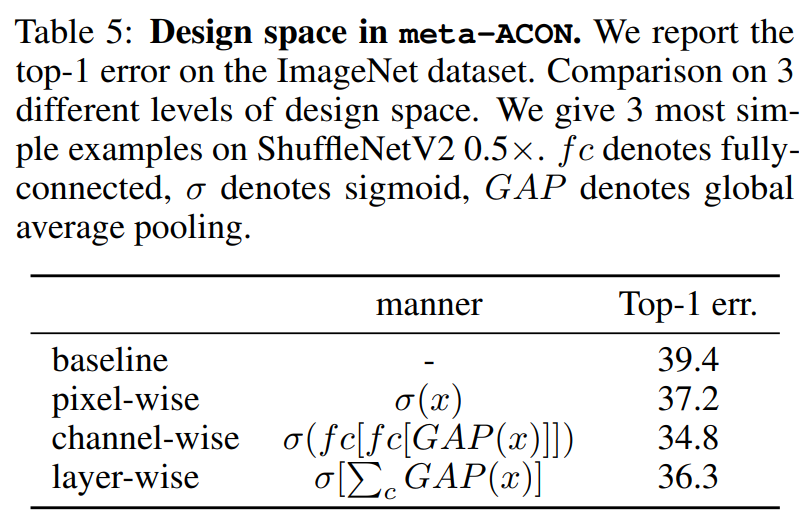# 小结¶